# Know Your Numbers: Number Worms

In this worksheet, students find the next number in a sequence given a simple rule.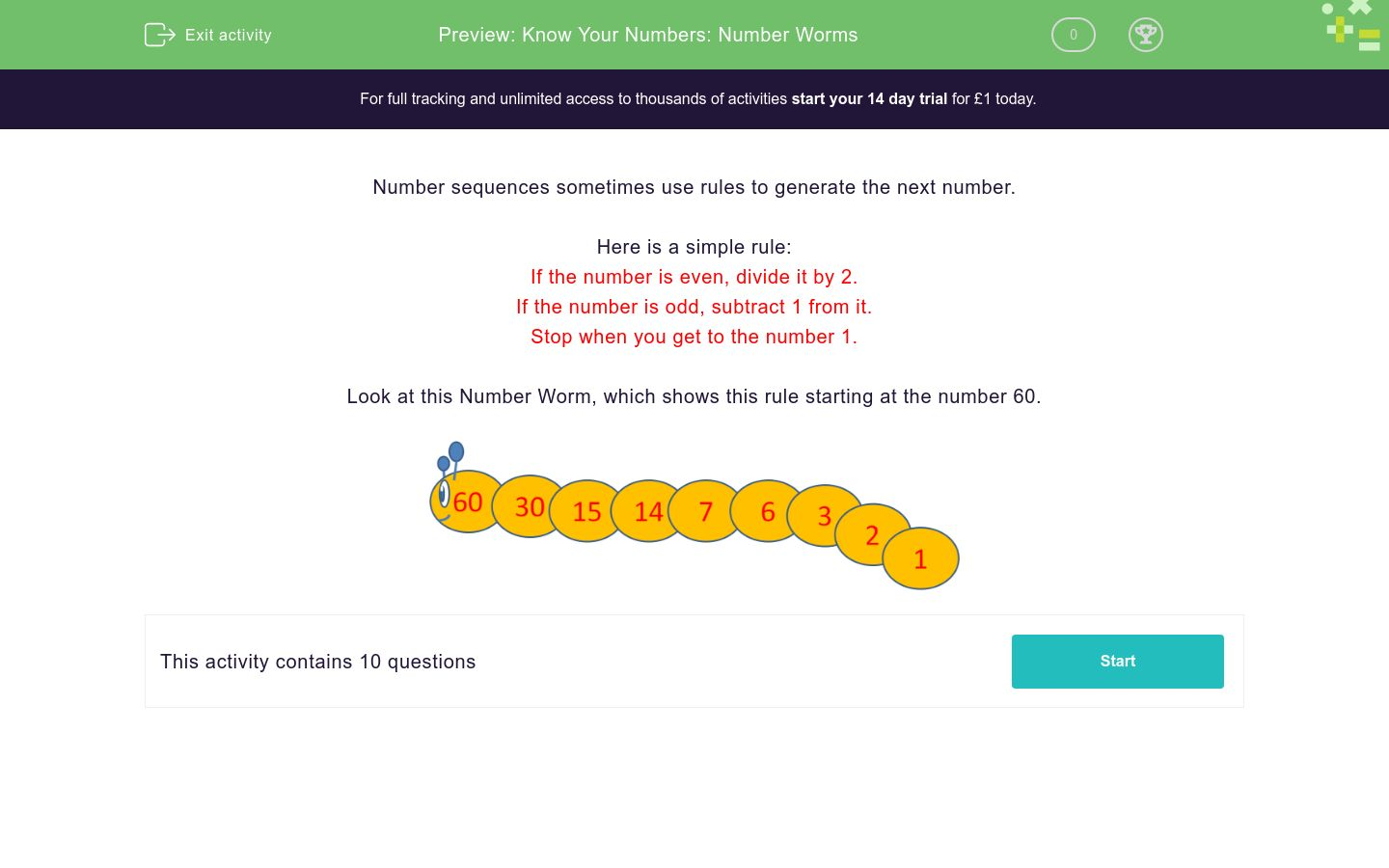Key stage:  KS 2

Curriculum topic:   Number: Number and Place Value

Curriculum subtopic:   Use Different Representations of Numbers

Difficulty level:### QUESTION 1 of 10

Number sequences sometimes use rules to generate the next number.

Here is a simple rule:

If the number is even, divide it by 2.

If the number is odd, subtract 1 from it.

Stop when you get to the number 1.

Look at this Number Worm, which shows this rule starting at the number 60.If the number is even, divide it by 2.

If the number is odd, subtract 1 from it.

Stop when you get to the number 1.

Work out the values of A, B and C in this number worm, using the rule above.## Column B

A
10
B
5
C
2

If the number is even, divide it by 2.

If the number is odd, subtract 1 from it.

Stop when you get to the number 1.

Work out the values of A, B and C in this number worm, using the rule above.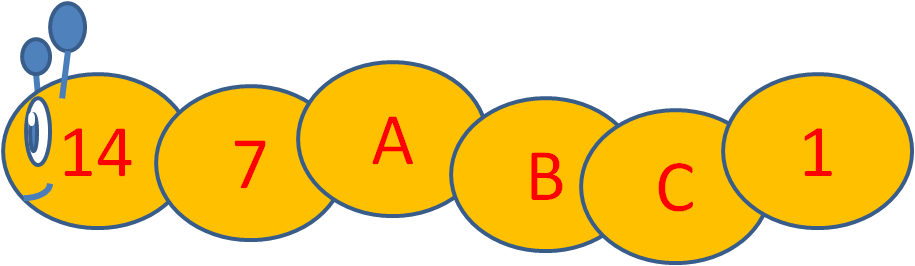## Column B

A
6
B
2
C
3

If the number is even, divide it by 2.

If the number is odd, subtract 1 from it.

Stop when you get to the number 1.

Work out the values of A, B and C in this number worm, using the rule above.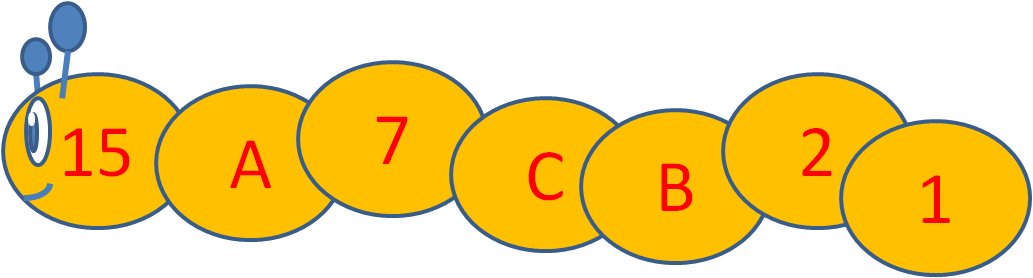## Column B

A
3
B
6
C
14

How many numbers will a Number Worm have starting at the number 6 ?

How many numbers will a Number Worm have starting at the number 16 ?

Will a Number Worm ever have two even numbers together?

Yes

No

Will a Number Worm ever have two odd numbers together?

Yes

No

Look at this Number Worm which follows the same rule as before.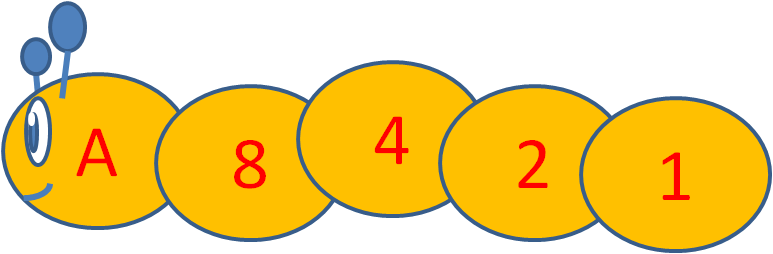Tick the values that A could be.

9

11

12

14

16

Look at this Number Worm which follows the same rule as before.Tick the values that A could be.

30

25

21

60

40

Look at this Number Worm which follows the same rule as before.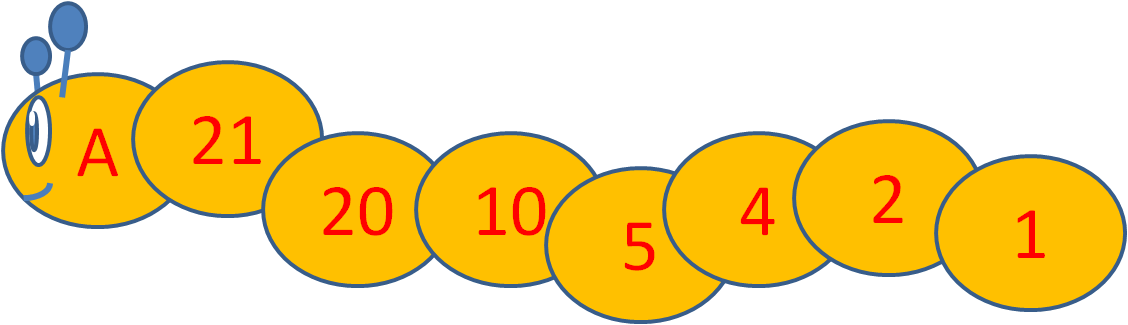Tick the values that A could be.

22

25

21

60

42

• Question 1

If the number is even, divide it by 2.

If the number is odd, subtract 1 from it.

Stop when you get to the number 1.

Work out the values of A, B and C in this number worm, using the rule above.## Column B

A
10
B
5
C
2
EDDIE SAYS
A) 20 divided by 2 = 10. B) 10 divided by 2 = 5. C) 4 divided by 2 = 2.
• Question 2

If the number is even, divide it by 2.

If the number is odd, subtract 1 from it.

Stop when you get to the number 1.

Work out the values of A, B and C in this number worm, using the rule above.## Column B

A
6
B
3
C
2
EDDIE SAYS
A) 7 - 1 = 6. B) 6 divided by 2 = 3. C) 3 - 1 = 2.
• Question 3

If the number is even, divide it by 2.

If the number is odd, subtract 1 from it.

Stop when you get to the number 1.

Work out the values of A, B and C in this number worm, using the rule above.## Column B

A
14
B
3
C
6
EDDIE SAYS
A) 15 - 1 = 14. B) 6 divided by 2 = 3. C) 7 - 1 = 6.
• Question 4

How many numbers will a Number Worm have starting at the number 6 ?

4
EDDIE SAYS
The Number worm starting with 6 is: 6, 3, 2, 1 The answer is 4 numbers.
• Question 5

How many numbers will a Number Worm have starting at the number 16 ?

5
EDDIE SAYS
The Number worm starting with 16 is: 16, 8, 4, 2, 1 The answer is 5 numbers.
• Question 6

Will a Number Worm ever have two even numbers together?

Yes
EDDIE SAYS
Yes, for example, a Number Worm starting at 16: 16, 8, 4, 2, 1 There are more than two even numbers together.
• Question 7

Will a Number Worm ever have two odd numbers together?

No
EDDIE SAYS
No, a Number Worm will never have two odd numbers together because every time there is an odd number, the rule says to subtract 1 from it, making it an even number.
• Question 8

Look at this Number Worm which follows the same rule as before.Tick the values that A could be.

9
16
EDDIE SAYS
A could be 9 because 9 - 1 = 8. A could be 16 because 16 divided by 2 = 8.
• Question 9

Look at this Number Worm which follows the same rule as before.Tick the values that A could be.

21
40
EDDIE SAYS
A could be 21 because 21 - 1 = 20. A could be 40 because 40 divided by 2 = 20.
• Question 10

Look at this Number Worm which follows the same rule as before.Tick the values that A could be.

42
EDDIE SAYS
A could be 42 because 42 divided by 2 = 21.
---- OR ----

Sign up for a £1 trial so you can track and measure your child's progress on this activity.

### What is EdPlace?

We're your National Curriculum aligned online education content provider helping each child succeed in English, maths and science from year 1 to GCSE. With an EdPlace account you’ll be able to track and measure progress, helping each child achieve their best. We build confidence and attainment by personalising each child’s learning at a level that suits them.

Get started